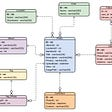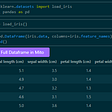# Data Visualisation using Matplotlib for beginners (Part 2)

In the previous blog, we saw how to plot simple line plots and do some formatting to make them look attractive. In case you haven't checked it out…check it now!

After the line plots let’s work with Scatter Plots.

# Scatter Plots

We will use the same data as we used for the line plot in the previous blog.

## 1. Make a simple scatter plot

We have three arrays x,y1, and y2.

`plt.scatter(x,y1)plt.scatter(x,y2)plt.show()`

## 2. Put Titles

The process remains the same as we did for the line plot

`plt.xlabel(“Time”)plt.ylabel(“Speed”)plt.title(“Speed v/s Time Curve”)`

## 3. Change the symbols

Till now you were getting dots on your plot. You can change this symbol using the marker parameter.

We can use symbols like stars and triangles.

`plt.scatter(x,y1, color=”red”, label=”A”, marker=”*”)plt.scatter(x,y2, color=”green”, label=”B”)plt.legend()plt.xlabel(“Time”)plt.ylabel(“Speed”)plt.title(“Speed v/s Time Scatter Plot”)plt.show`
`plt.scatter(x,y1, color=”red”, label=”A”, marker=”^”)plt.scatter(x,y2, color=”green”, label=”B”)plt.legend()plt.xlabel(“Time”)plt.ylabel(“Speed”)plt.title(“Speed v/s Time Scatter Plot”)plt.show`

## 4. Adjust the size of any plot

We can adjust the size of any plot with help of plt. figure(figsize=(2,2)). You can give any dimensions in place of (2,2), and see the output.

`plt.figure(figsize=(2,2))plt.scatter(x,y1, color=”red”, label=”A”, marker=”^”)plt.scatter(x,y2, color=”green”, label=”B”)plt.legend()plt.xlabel(“Time”)plt.ylabel(“Speed”)plt.title(“Speed v/s Time Scatter Plot”)plt.show`
`plt.figure(figsize=(10,5))`
`plt.figure(figsize=(8,2))`

You can choose the best fit as per your need. Keep experimenting with more parameters.

## More from Shatakshi Singh

Love podcasts or audiobooks? Learn on the go with our new app.

## Choose the right database for your job## How to improve your Scala programming skills?## Develop Your Own Operating System## 31,536,000 Seconds of Code — To Pastures New## Bluehost Reviews — 10 Pros & Cons You Should Know Now## PHPUnit and Xdebug — up and running## How to be more productive by focusing on the right things (for programmers)## Protecting Private Data using PII Analyses in Python## Hello Mito — A Beginner Friendly Pandas Tool## How to calculate Pareto distribution and Zipf’s law in Python